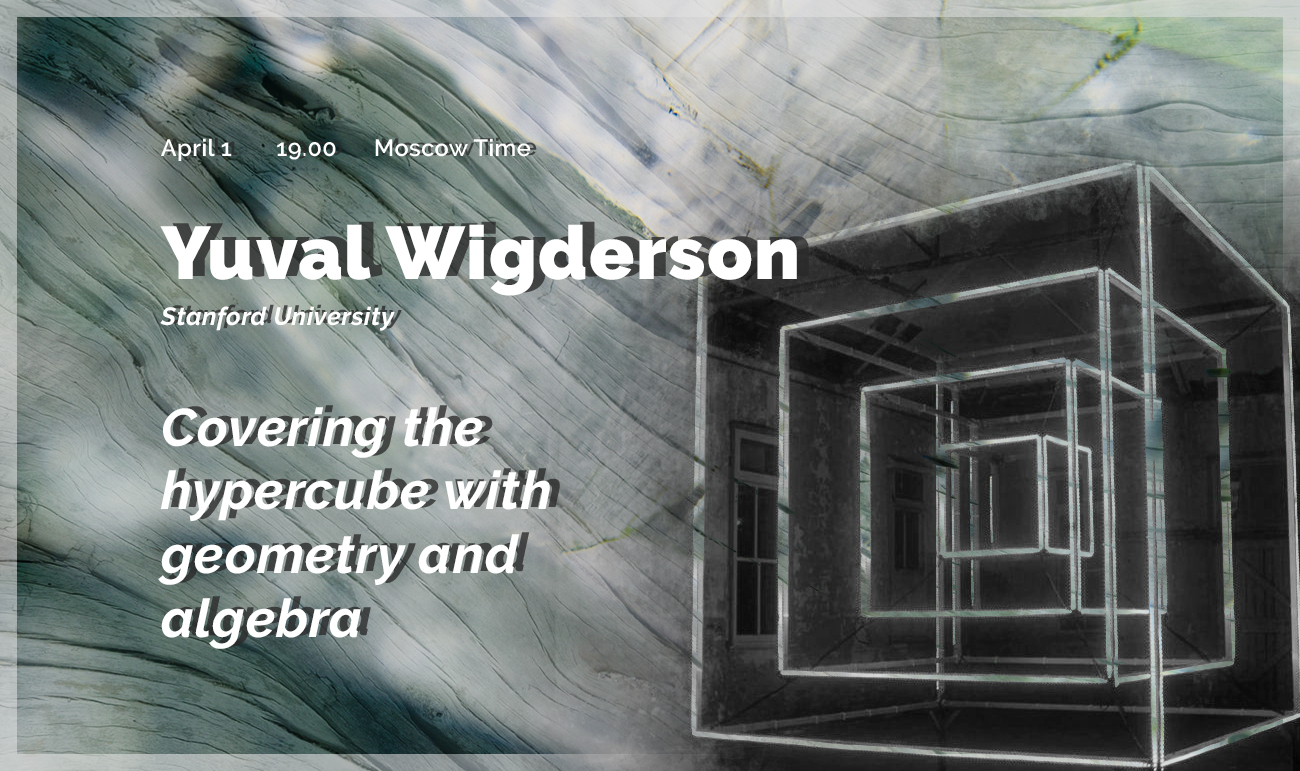# Yuval Wigderson “Covering the hypercube with geometry and algebra” | Big SeminarApril 1, 2021
19.00 MSK (UTC +3)
Talk on Big Seminar

# Yuval Wigderson "Covering the hypercube with geometry and algebra"

Yuval Wigderson from Stanford University gave the talk "Covering the hypercube with geometry and algebra" on the labs' Big Seminar.

The talk will be held in zoom
Password: first 6 decimal places of $\pi$ after the decimal point

You can also write to Alexander Polyanskii (alexander.polyanskii@yandex.ru) or to Maksim Zhukovskii (zhukmax@gmail.com) if you want to be added to mailing list.

Abstract:

What is the smallest number of hyperplanes needed to cover the vertices of the hypercube $\{0, 1\}^n$? At least two hyperplanes are needed, and since we may take the parallel hyperplanes $x_1=0$ and $x_1=1$, we see that two hyperplanes suffice.

Although the question above is not particularly interesting, there are many interesting variants of it, with connections to discrete geometry, infinite Ramsey theory, theoretical computer science, extremal combinatorics, and beyond. One such question is the following: how many hyperplanes are needed to cover all vertices of the hypercube except the origin, while not covering the origin? Here, Alon and Füredi proved that at least $n$ hyperplanes are needed, and it is easy to see that $n$ hyperplanes also suffice.

Although these problems are geometric in nature, all known proofs of the Alon–Füredi theorem use algebraic techniques, relating the hyperplane covering problem to a polynomial covering problem and then resolving this latter problem. Miraculously, the geometric problem and its algebraic relaxation turn out to have the same answer. In this talk, I will survey some results and questions about covers of the hypercube, and then discuss a recent result showing that for a natural higher-multiplicity version of the Alon–Füredi theorem proposed by Clifton and Huang, the geometric and algebraic problems (probably) have very different answers.

Joint work with Lisa Sauermann.

Slides

## Watch the video:

Everyone is invited to attend. The language of the lecture is English. The event is aimed at master and graduate students, as well as researchers in the field of combinatorics.